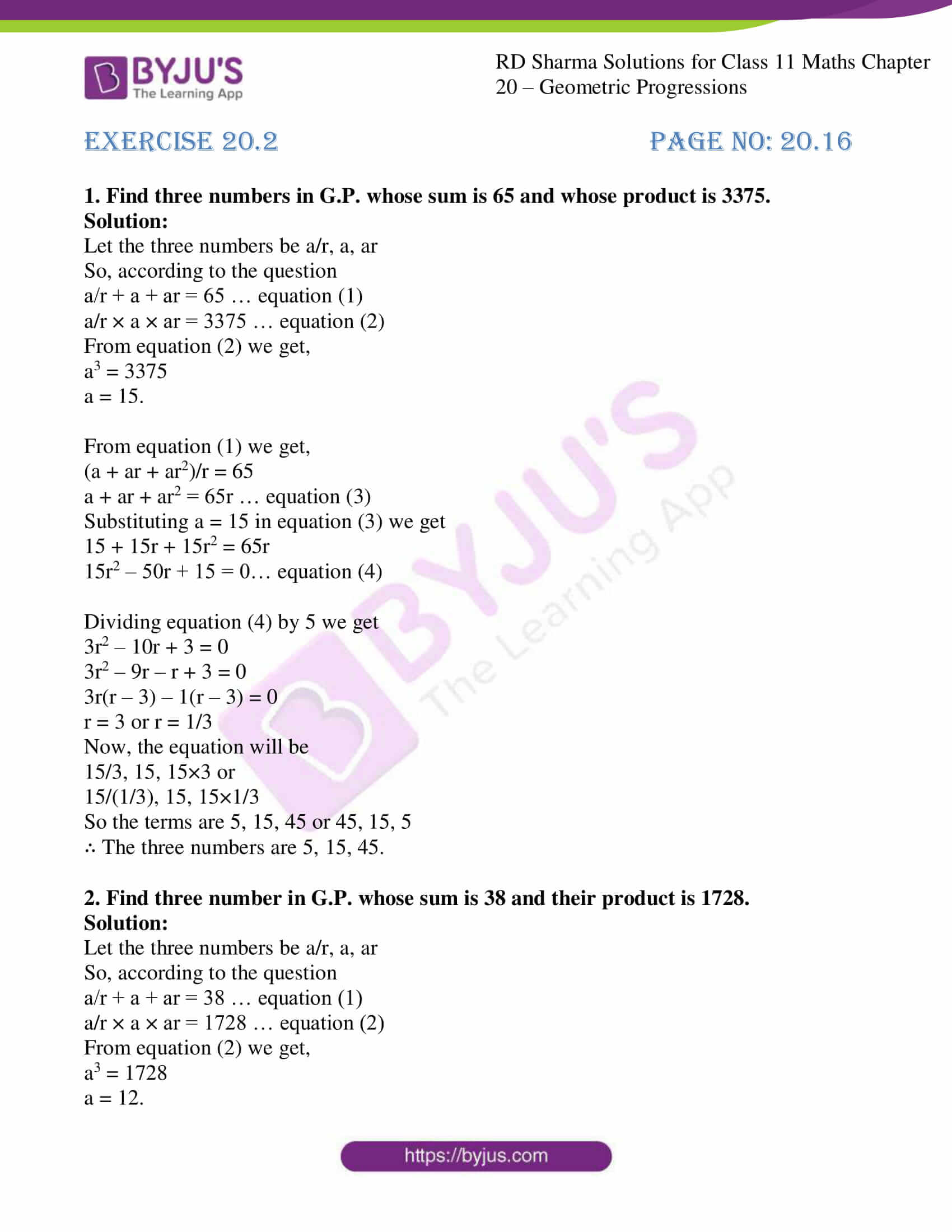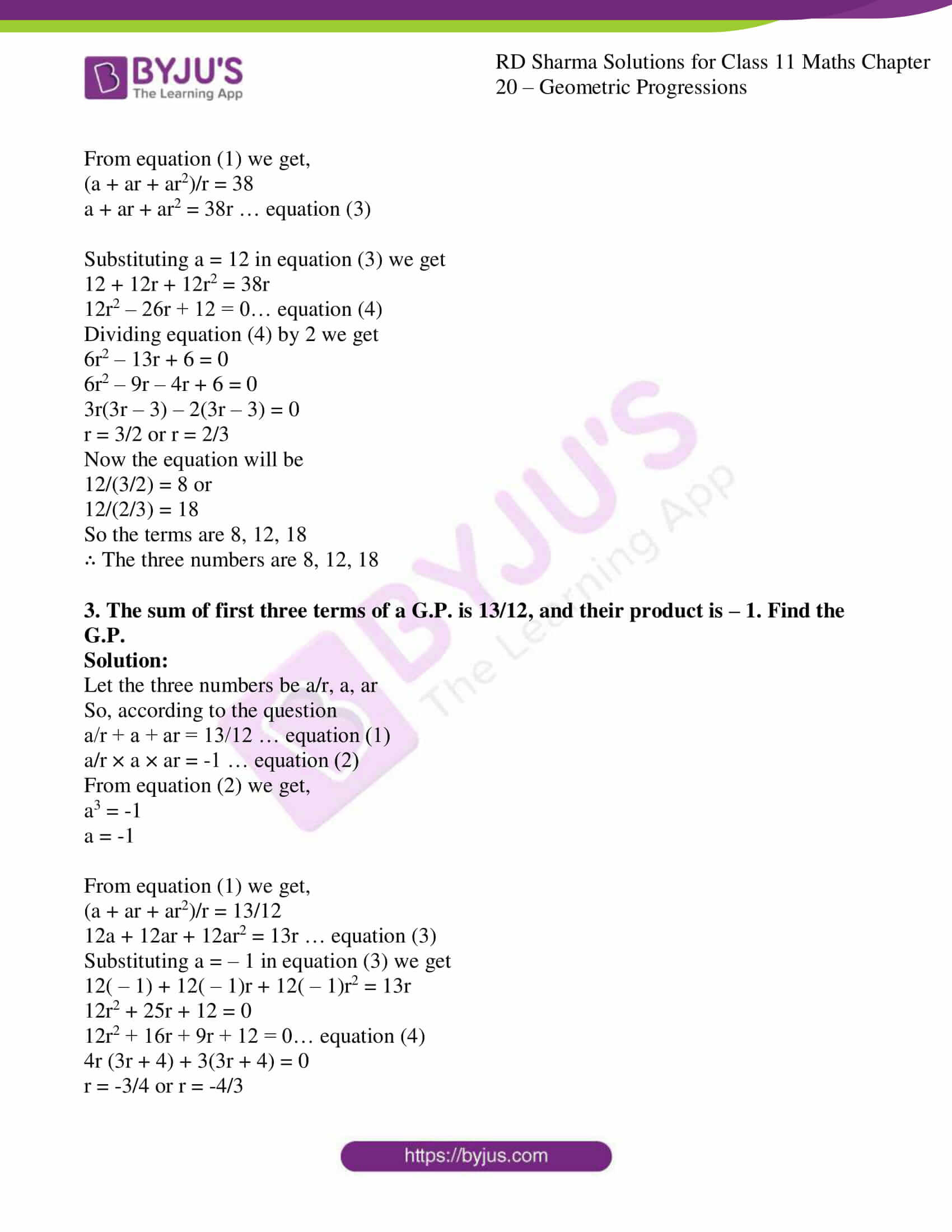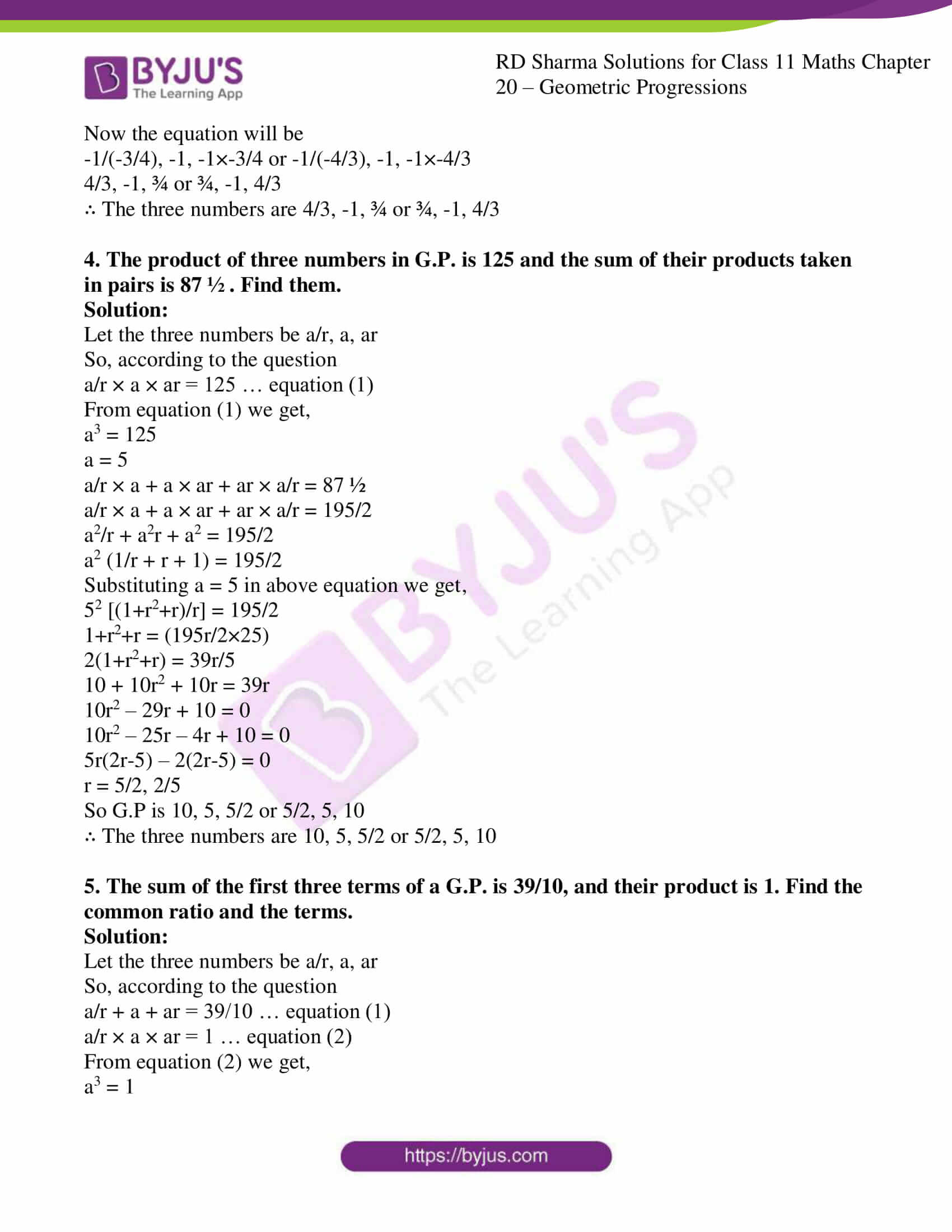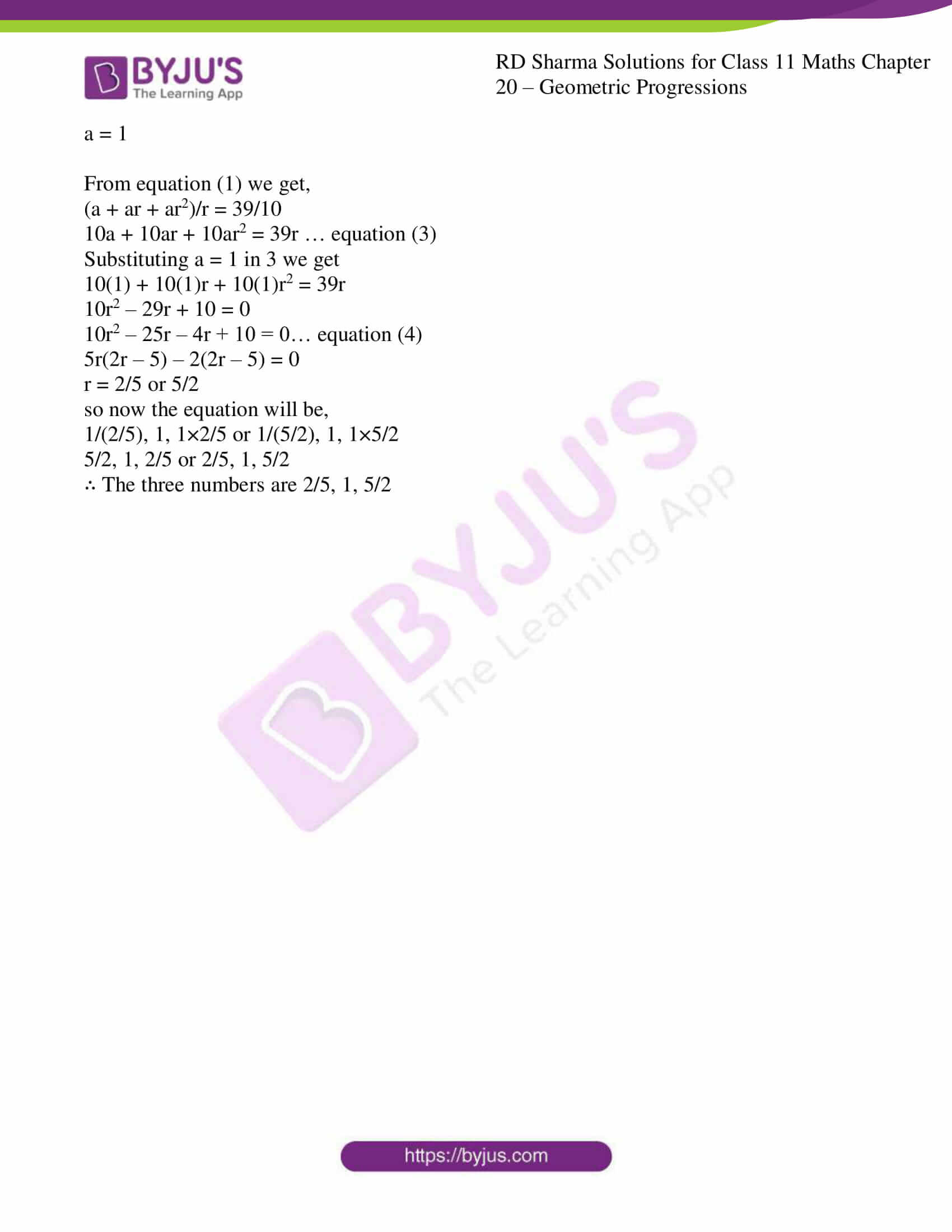# RD Sharma Solutions Class 11 Geometric Progressions Exercise 20.2

Sometimes it is required to select a finite number of terms in G.P. If the product of the numbers is not given, then the numbers are taken as a, ar… In this exercise, we mainly study problems based on a selection of terms in G.P. Examples are provided, which illustrate the application of a selection of terms. Experts at BYJU’S have solved the exercise problems using shortcut techniques to help students increase the speed of solving the problems. Students can also refer to RD Sharma Class 11 Maths Solutions and by practising regularly, they can achieve their goals of scoring high marks. These solutions are available in the pdf format, students can download them and can learn offline.

## Download the pdf of RD Sharma Solutions for Class 11 Maths Exercise 20.2 Chapter 20 – Geometric Progression### Access answers to RD Sharma Solutions for Class 11 Maths Exercise 20.2 Chapter 20 – Geometric Progression

1. Find three numbers in G.P. whose sum is 65 and whose product is 3375.

Solution:

Let the three numbers be a/r, a, ar

So, according to the question

a/r + a + ar = 65 … equation (1)

a/r × a × ar = 3375 … equation (2)

From equation (2) we get,

a3 = 3375

a = 15.

From equation (1) we get,

(a + ar + ar2)/r = 65

a + ar + ar2 = 65r … equation (3)

Substituting a = 15 in equation (3) we get

15 + 15r + 15r2 = 65r

15r2 – 50r + 15 = 0… equation (4)

Dividing equation (4) by 5 we get

3r2 – 10r + 3 = 0

3r2 – 9r – r + 3 = 0

3r(r – 3) – 1(r – 3) = 0

r = 3 or r = 1/3

Now, the equation will be

15/3, 15, 15×3 or

15/(1/3), 15, 15×1/3

So the terms are 5, 15, 45 or 45, 15, 5

∴ The three numbers are 5, 15, 45.

2. Find three number in G.P. whose sum is 38 and their product is 1728.

Solution:

Let the three numbers be a/r, a, ar

So, according to the question

a/r + a + ar = 38 … equation (1)

a/r × a × ar = 1728 … equation (2)

From equation (2) we get,

a3 = 1728

a = 12.

From equation (1) we get,

(a + ar + ar2)/r = 38

a + ar + ar2 = 38r … equation (3)

Substituting a = 12 in equation (3) we get

12 + 12r + 12r2 = 38r

12r2 – 26r + 12 = 0… equation (4)

Dividing equation (4) by 2 we get

6r2 – 13r + 6 = 0

6r2 – 9r – 4r + 6 = 0

3r(3r – 3) – 2(3r – 3) = 0

r = 3/2 or r = 2/3

Now the equation will be

12/(3/2) = 8 or

12/(2/3) = 18

So the terms are 8, 12, 18

∴ The three numbers are 8, 12, 18

3. The sum of first three terms of a G.P. is 13/12, and their product is – 1. Find the G.P.

Solution:

Let the three numbers be a/r, a, ar

So, according to the question

a/r + a + ar = 13/12 … equation (1)

a/r × a × ar = -1 … equation (2)

From equation (2) we get,

a3 = -1

a = -1

From equation (1) we get,

(a + ar + ar2)/r = 13/12

12a + 12ar + 12ar2 = 13r … equation (3)

Substituting a = – 1 in equation (3) we get

12( – 1) + 12( – 1)r + 12( – 1)r2 = 13r

12r2 + 25r + 12 = 0

12r2 + 16r + 9r + 12 = 0… equation (4)

4r (3r + 4) + 3(3r + 4) = 0

r = -3/4 or r = -4/3

Now the equation will be

-1/(-3/4), -1, -1×-3/4 or -1/(-4/3), -1, -1×-4/3

4/3, -1, ¾ or ¾, -1, 4/3

∴ The three numbers are 4/3, -1, ¾ or ¾, -1, 4/3

4. The product of three numbers in G.P. is 125 and the sum of their products taken in pairs is 87 ½ . Find them.

Solution:

Let the three numbers be a/r, a, ar

So, according to the question

a/r × a × ar = 125 … equation (1)

From equation (1) we get,

a3 = 125

a = 5

a/r × a + a × ar + ar × a/r = 87 ½

a/r × a + a × ar + ar × a/r = 195/2

a2/r + a2r + a2 = 195/2

a2 (1/r + r + 1) = 195/2

Substituting a = 5 in above equation we get,

52 [(1+r2+r)/r] = 195/2

1+r2+r = (195r/2×25)

2(1+r2+r) = 39r/5

10 + 10r2 + 10r = 39r

10r2 – 29r + 10 = 0

10r2 – 25r – 4r + 10 = 0

5r(2r-5) – 2(2r-5) = 0

r = 5/2, 2/5

So G.P is 10, 5, 5/2 or 5/2, 5, 10

∴ The three numbers are 10, 5, 5/2 or 5/2, 5, 10

5. The sum of the first three terms of a G.P. is 39/10, and their product is 1. Find the common ratio and the terms.

Solution:

Let the three numbers be a/r, a, ar

So, according to the question

a/r + a + ar = 39/10 … equation (1)

a/r × a × ar = 1 … equation (2)

From equation (2) we get,

a3 = 1

a = 1

From equation (1) we get,

(a + ar + ar2)/r = 39/10

10a + 10ar + 10ar2 = 39r … equation (3)

Substituting a = 1 in 3 we get

10(1) + 10(1)r + 10(1)r2 = 39r

10r2 – 29r + 10 = 0

10r2 – 25r – 4r + 10 = 0… equation (4)

5r(2r – 5) – 2(2r – 5) = 0

r = 2/5 or 5/2

so now the equation will be,

1/(2/5), 1, 1×2/5 or 1/(5/2), 1, 1×5/2

5/2, 1, 2/5 or 2/5, 1, 5/2

∴ The three numbers are 2/5, 1, 5/2